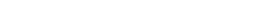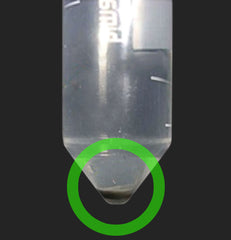# Difference between swing-out (bucket) and fixed angle rotors

### Feb 27, 2017

First of all, due to the confusions in the names of the rotors, let’s explain what are the swing out and fixed angle rotors:
1. Swing-out rotor (also known as bucket rotor, rotor with variable angle) – is the rotor, in which tubes are located in the buckets (tube-holders) and those buckets are not rigidly attached to the rotor with certain angle. Instead buckets are attached to the rotor in a way, that allows them to spin horizontally (angle relative to the axis of rotation - 90 degrees). Fixed angle rotor – is the rotor, which holds tubes in the exact same fixed angle all the time, usually 45 degrees.
2. Main difference between above-mentioned types of rotor is connected with “working angle” (angle relative to the axis of rotation). The force that affects the centrifuged sample (RCF, relative centrifugal force) is directed exactly perpendicular to the axis of rotation of the rotor. Thus, the sample is divided strictly in the direction of RCF. If bucket rotor is used – the RCF is directed through the center of the tubePic. 1.The sample, divided with the use of the swing-out (bucket) rotor. It is clearly visible, that precipitate is located in the center of the tube. In case of using fixed angle rotor, the direction of separation is 45 degrees relative to the axis of rotation.Pic. 2 The sample, divided with the use of the fixed angle rotor. It is clearly visible, that precipitate is located on the walls of the tube. The pictures shows, that use of the swing out (bucket) rotor is more convenient way to separate the samples, due to the fact that it simplifies the handling and storage of the sample after centrifugation